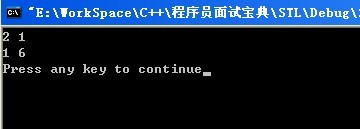C++模板

1.模板的概念。

//函数1.

int max(int x,int y);

{return(x>y)?x:y ;}

//函数2.

float max( float x,float y){
return (x>y)? x:y ;}

//函数3.

double max(double x,double y)
{return (c>y)? x:y ;}

2.   函数模板的写法

Template <class或者也可以用typename T>

{//函数定义体 }

//Test.cpp

#include<iostream>

using std::cout;

using std::endl;

//声明一个函数模版,用来比较输入的两个相同数据类型的参数的大小，class也可以被typename代替，

//T可以被任何字母或者数字代替。

template <class T>

T min(T x,T y)

{ return(x<y)?x:y;}

void main( )

{

int n1=2,n2=10;

double d1=1.5,d2=5.6;

cout<< "较小整数:"<<min(n1,n2)<<endl;

cout<< "较小实数:"<<min(d1,d2)<<endl;

system("PAUSE");

}3.类模板的写法

Template < class或者也可以用typename T >

class类名｛
／／类定义．．．．．．
｝；

// ClassTemplate.h

#ifndef ClassTemplate_HH

#define ClassTemplate_HH

template<typename T1,typename T2>

class myClass{

private:

T1 I;

T2 J;

public:

myClass(T1 a, T2 b);//Constructor

void show();

};

//这是构造函数

//注意这些格式

template <typename T1,typename T2>

myClass<T1,T2>::myClass(T1 a,T2 b):I(a),J(b){}

//这是void show();

template <typename T1,typename T2>

void myClass<T1,T2>::show()

{

cout<<"I="<<I<<", J="<<J<<endl;

}

#endif

// Test.cpp

#include<iostream>

#include"ClassTemplate.h"

using std::cout;

using std::endl;

void main()

{

myClass<int,int> class1(3,5);

class1.show();

myClass<int,char> class2(3,'a');

class2.show();

myClass<double,int> class3(2.9,10);

class3.show();

system("PAUSE");

}

4.非类型模版参数

template<typename T,int MAXSIZE>

class Stack{

Private:

T elems[MAXSIZE];

};

Int main()

{

Stack<int, 20> int20Stack;

Stack<int, 40> int40Stack;

};

5.使用模板类型

template <typename T>

void print(T v)
{
T::iterator itor;
for (itor = v.begin(); itor != v.end(); ++itor)
{
cout << *itor << " ";
}
cout << endl;
}

void main(int argc, char **argv){

list<int> l;
l.push_back(1);
l.push_front(2);
if(!l.empty())
print(l);
vector<int> vec;
vec.push_back(1);
vec.push_back(6);
if(!vec.empty())
print(vec);
}左值变换
修饰字转换
派生类到基类的转换
见《C++ Primer》（[注2]，P500）对此主题的完备讨论。

6. 模板的特化

template<> // 这代表了下面是一个模板函数
long max<long>( long a, long b ) // 对于 vc 来说，这里的 <long> 是可以省略的
{
return a > b ? b : a;
}

template<T1, T2>

class MyClass;

template<T1, T2>

class MyCalss<int, T2>//偏特化

7. 仿函数

void dosome( int i )

1　仿函数不是函数，它是个类；
2　仿函数重载了()运算符，使得它的对你可以像函数那样子调用(代码的形式好像是在调用比如：
struct DoSome
{
void operator()( int i );
}
DoSome dosome;

函数指针指向的对象。
typedef void( *DoSomePtr )( int );
typedef void( DoSome )( int );
DoSomePtr *ptr=&func;
DoSome& dosome=*ptr;

dosome(5); // 这里又和函数调用一模一样了。

8. 仿函数的用处

STL 模板库中，大量使用了这种技巧，来实现库的“灵活”。

for_each, 它的源代码大致如下：
template< typename Iterator, typename Functor >
void for_each( Iterator begin, Iterator end, Fucntor func )
{
for( ; begin!=end; begin++ )
func( *begin );
}

#### 最新留言

[***.219.124.196]2023年09月10日 16时53分07秒

## 关于作者喝酒易醉，品茶养心，人生如梦，品茶悟道，何以解忧？唯有杜康！
-- 愿君每日到此一游！